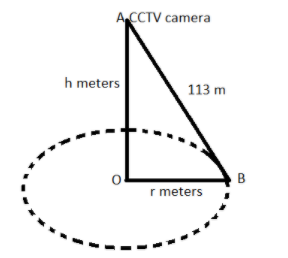Courses
Courses for Kids
Free study material
Free LIVE classes
MoreLIVE
Join Vedantu’s FREE Mastercalss

# For traffic control, a CCTV camera is fixed on a straight and vertical pole. The camera can see 113m distance straight from the top. If the area visible by the camera around the pole is 39424${m^2}$, then find the height of the pole.Verified
335.1k+ views
Hint: In this particular question use the concept that the area visible by the camera around the pole is always in circular shape, so equate the given area to the area of the circle and calculate the radius of the circle, later on in the solution use the concept of Pythagoras theorem so use these concepts to reach the solution of the question.Let OA be the vertical pole as shown in the above figure, at point A, CCTV camera is fixed and the reach of the CCTV camera is up to 113m as shown in the above figure.
Let the reach is up to point B, so AB = 113m (see figure).
Let the height of the vertical pole be h meters.
Therefore, OA = h meters.
Now it is given that the area visible by the camera around the pole is 39424${m^2}$.
As we all know that the visible area is always around a circle as shown in the figure, and the area of the circle is given as $\pi {r^2}$ square units, where r is the radius of the circle.
Therefore, $\pi {r^2} = 39424$
$\Rightarrow {r^2} = \dfrac{{39424}}{\pi } = \dfrac{{39424}}{{\dfrac{{22}}{7}}} = 12544 = {\left( {112} \right)^2}$, $\left[ {\because \pi = \dfrac{{22}}{7}} \right]$
$\Rightarrow r = 112$Meters.
As the pole is vertical so it makes a 90 degree with the radius of the circle.
So in triangle OAB apply Pythagoras theorem we have,
Now as we know that according to the Pythagoras theorem that in a right triangle the sum of the square of the base and perpendicular is equal to the square of the hypotenuse.
$\Rightarrow {\left( {{\text{base}}} \right)^2} + {\left( {{\text{perpendicular}}} \right)^2} = {\left( {{\text{hypotenuse}}} \right)^{^2}}$................. (1)
Now in triangle OAB, base = OB, perpendicular = OA and hypotenuse = AB
Now from equation (1) we have,
$\Rightarrow {\left( {{\text{OB}}} \right)^2} + {\left( {{\text{OA}}} \right)^2} = {\left( {{\text{AB}}} \right)^{^2}}$
Now substitute the values we have,
$\Rightarrow {\left( {{\text{112}}} \right)^2} + {\left( {\text{h}} \right)^2} = {\left( {{\text{113}}} \right)^{^2}}$
$\Rightarrow {\left( {\text{h}} \right)^2} = {\left( {{\text{113}}} \right)^{^2}} - {\left( {{\text{112}}} \right)^2} = 225 = {\left( {15} \right)^2}$
$\Rightarrow h = 15$ Meters.
So the height of the vertical pole is 15 meters.

Note: Whenever we face such types of questions the key concept we have to remember is that always recall the formula of the area of the circle which is given as $\pi {r^2}$ square units, and always recall the definition of the Pythagoras theorem which is stated above then simplify substitute the values as above and simplify we will get the required height of the pole.
Last updated date: 26th Sep 2023
Total views: 335.1k
Views today: 4.35k